This site uses cookies. By continuing to browse the ConceptDraw site you are agreeing to our Use of Site Cookies.
ConceptDraw Samples | Graphs and charts — Histogram

# Histograms

Samples of histograms are created using ConceptDraw DIAGRAM diagramming and vector drawing software and the Histograms solution.

ConceptDraw DIAGRAM provides export of vector graphic multipage documents into multiple file formats: vector graphics (SVG, EMF, EPS), bitmap graphics (PNG, JPEG, GIF, BMP, TIFF), web documents (HTML, PDF), PowerPoint presentations (PPT), Adobe Flash (SWF).

## Tutorials and Solutions:

Video Tutorials: ConceptDraw Solution Park

Solutions: Histograms for ConceptDraw DIAGRAM

## Sample 1: Beetles in the River Thames

Histograms sample: Beetles in the river Thames.

This example is created using ConceptDraw DIAGRAM diagramming software enhanced with Histograms solution from ConceptDraw Solution Park.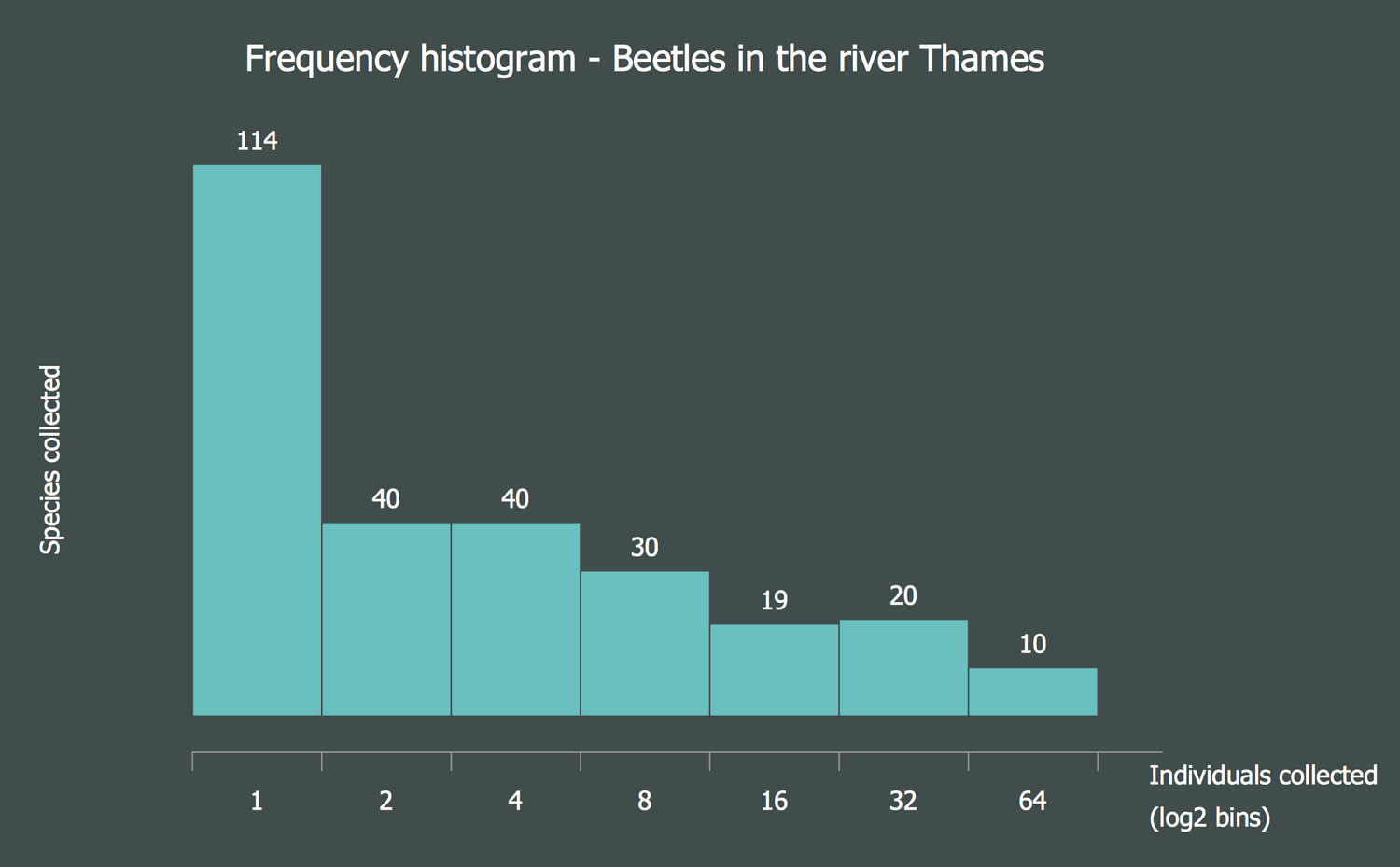## Sample 2: Black Cherry Tree Histogram

Histograms sample: Black cherry tree histogram.

This example is created using ConceptDraw DIAGRAM diagramming software enhanced with Histograms solution from ConceptDraw Solution Park.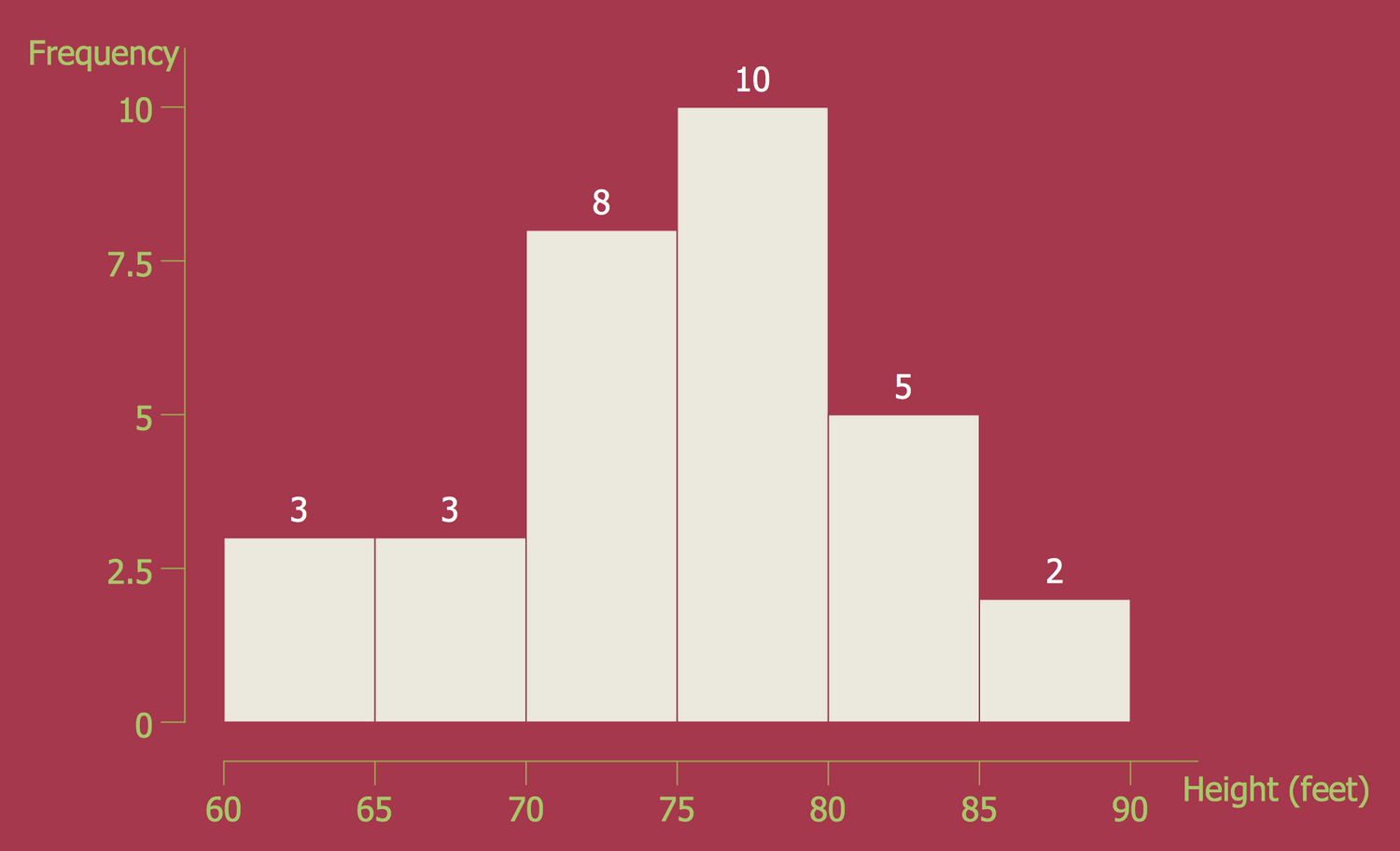## Sample 3: Amino Acid Length Distribution 2010

Histograms sample: Amino acid length distribution 2010.

This example is created using ConceptDraw DIAGRAM diagramming software enhanced with Histograms solution from ConceptDraw Solution Park.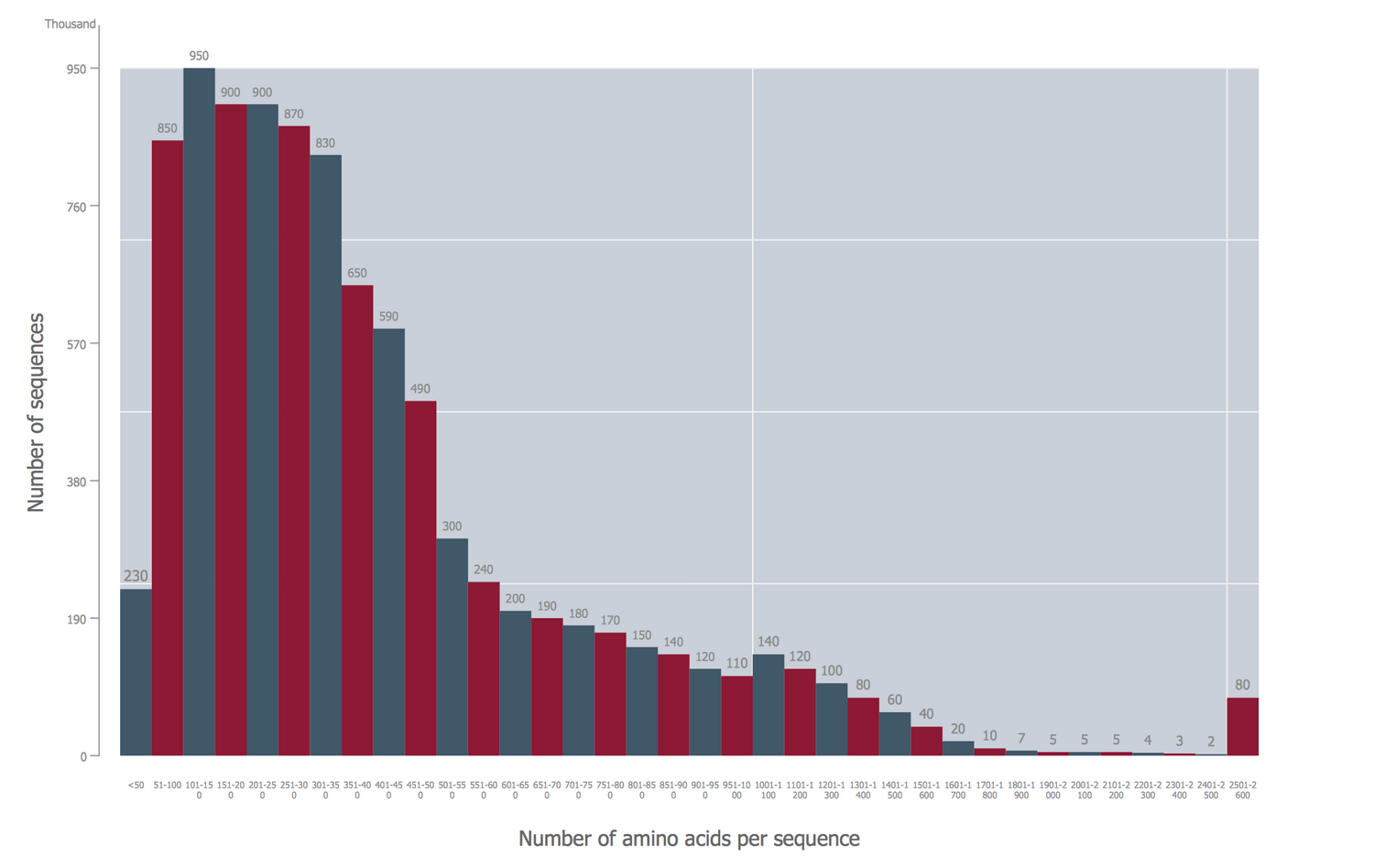## Sample 4: FICO® Scores

Histograms sample: FICO® scores.

This example is created using ConceptDraw DIAGRAM diagramming software enhanced with Histograms solution from ConceptDraw Solution Park.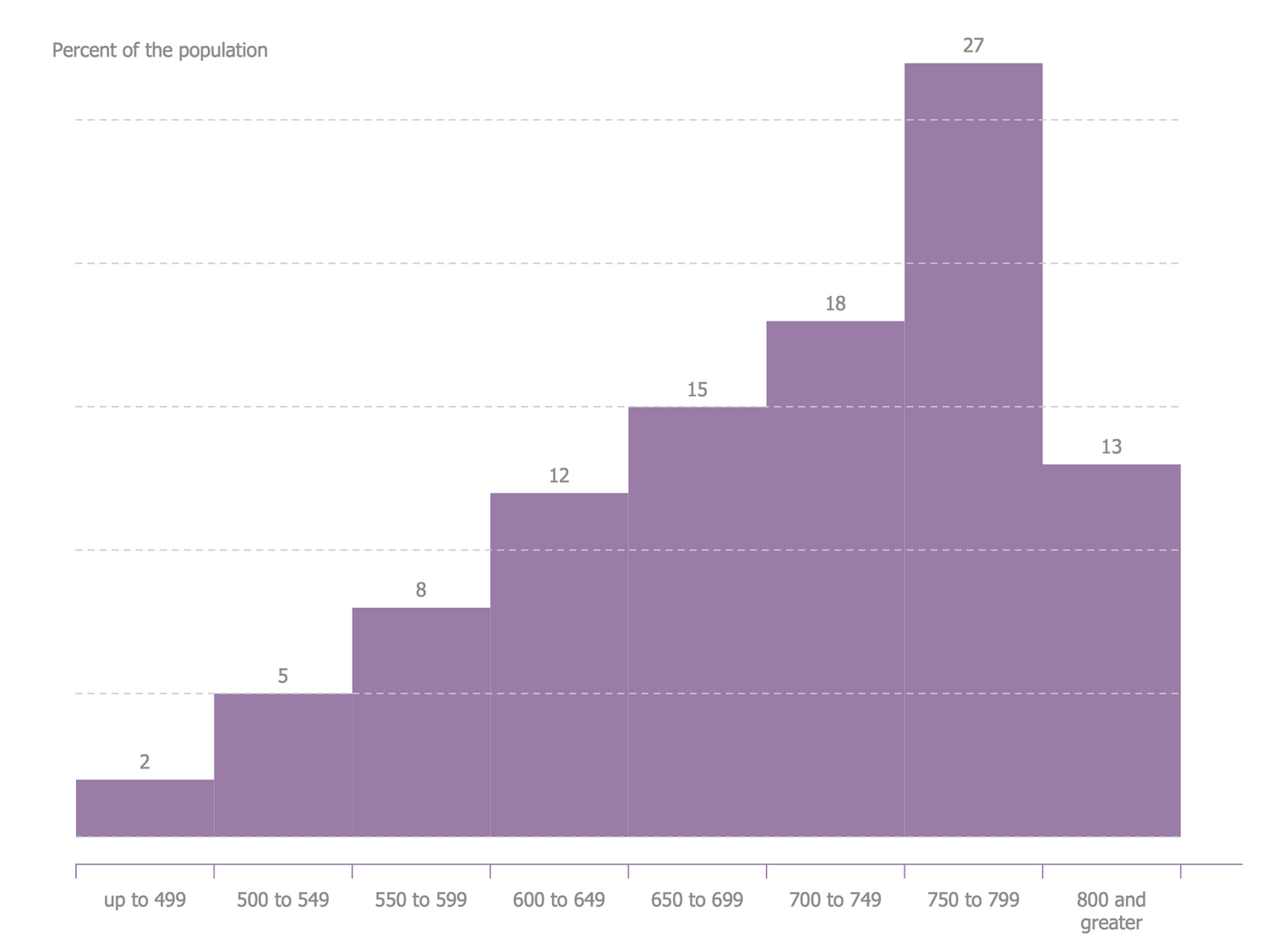## Sample 5: Page Load Time

This example is created using ConceptDraw DIAGRAM diagramming software enhanced with Histograms solution from ConceptDraw Solution Park.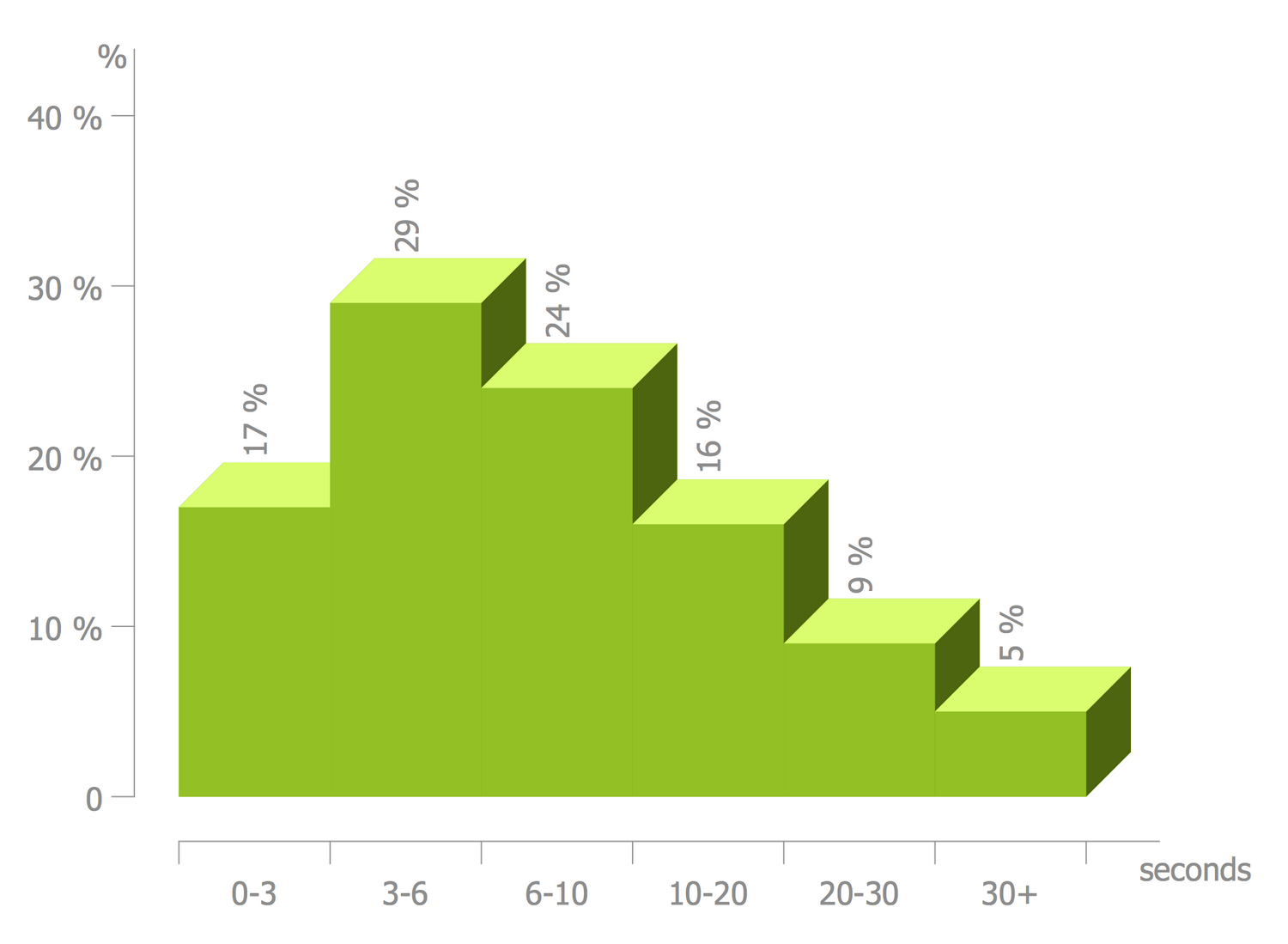## Sample 6: Age of Presidental Ascention

Histograms sample: Age of presidental ascention.

This example is created using ConceptDraw DIAGRAM diagramming software enhanced with Histograms solution from ConceptDraw Solution Park.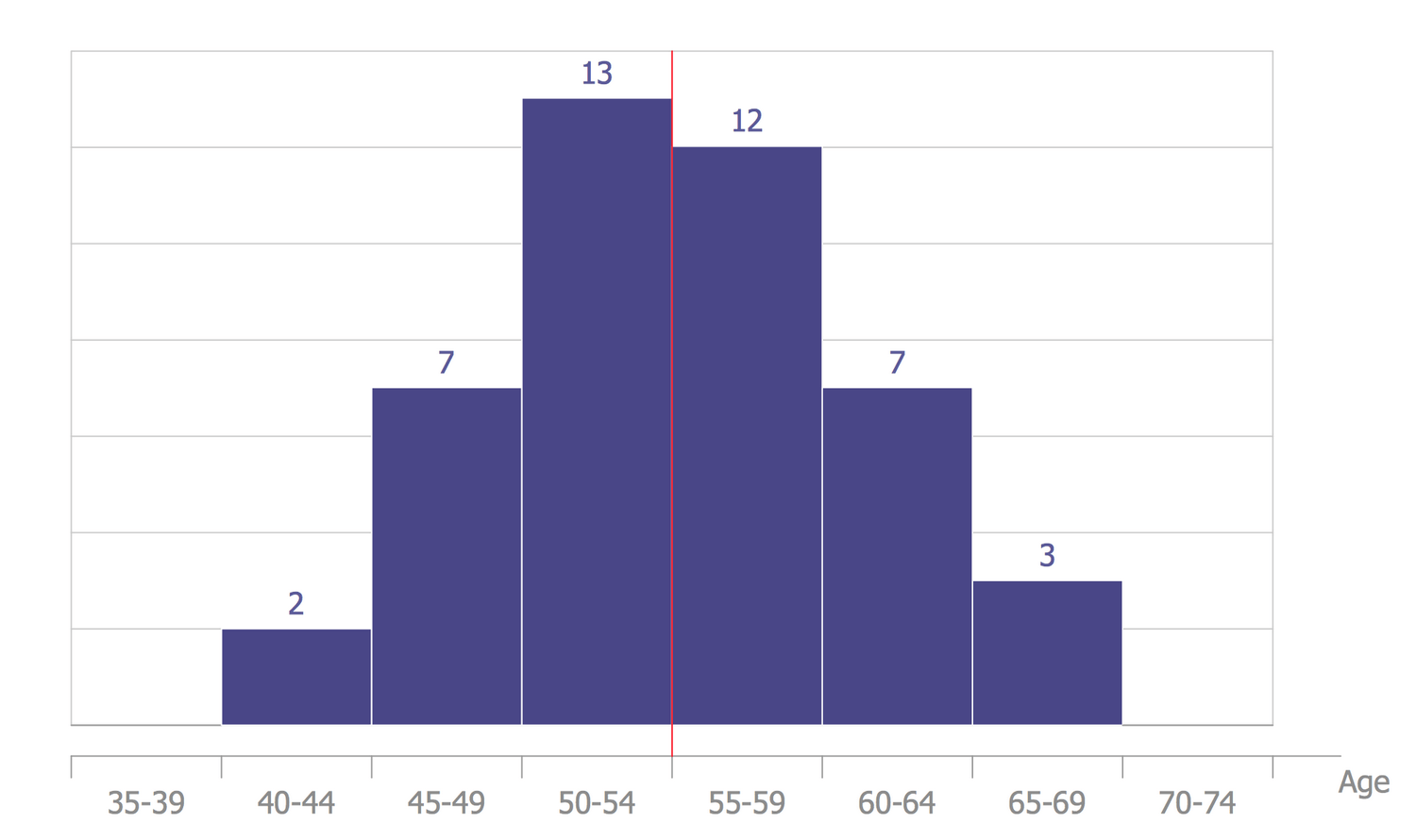## Sample 7: Mass Distribution of Food Rations

Histograms sample: Mass Distribution of Food Rations.

This example is created using ConceptDraw DIAGRAM diagramming software enhanced with Histograms solution from ConceptDraw Solution Park.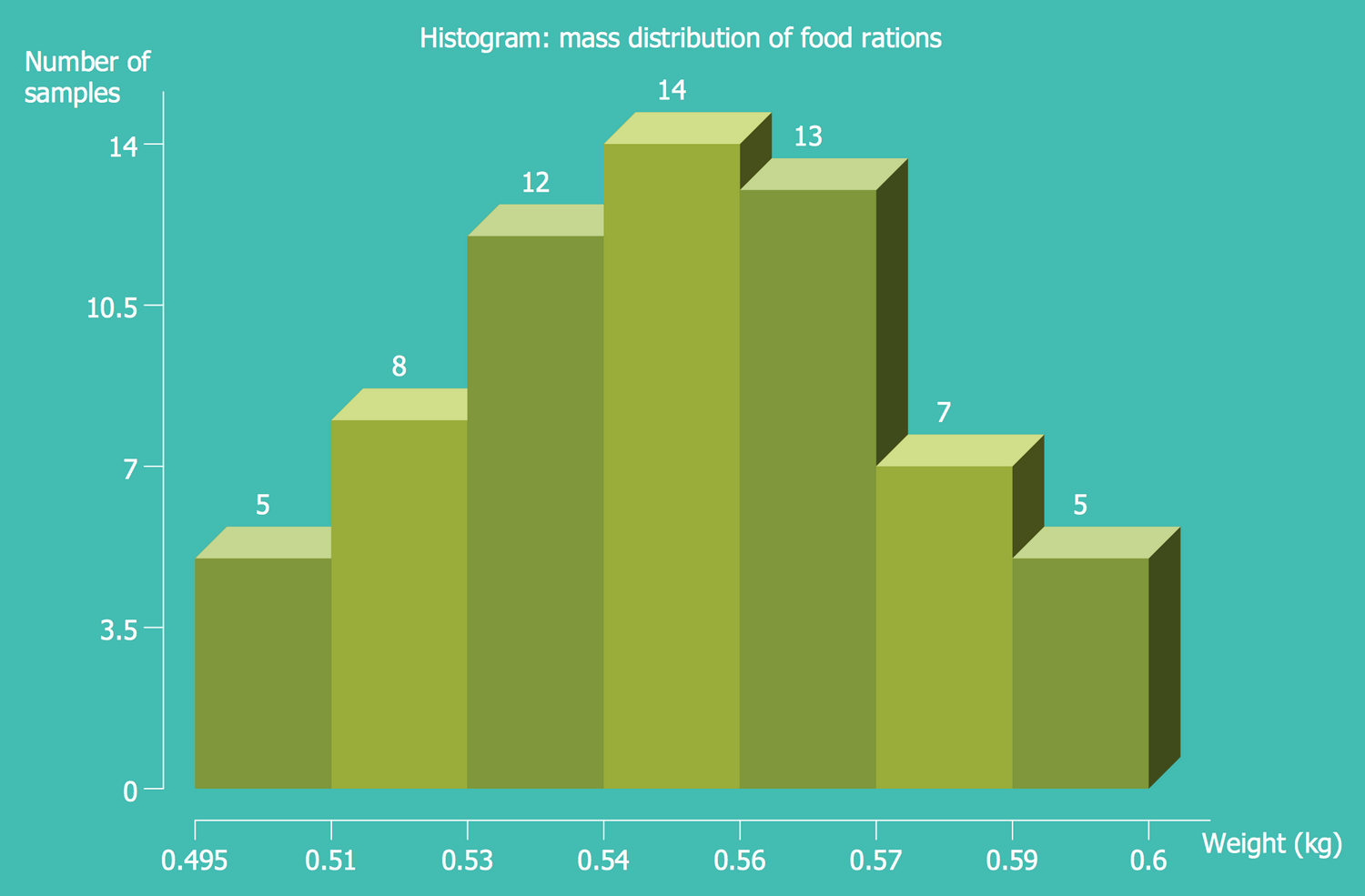## Sample 8: Mass Distribution of the Culinary Rations

Histograms sample: Mass Distribution of the Culinary Rations.

This example is created using ConceptDraw DIAGRAM diagramming software enhanced with Histograms solution from ConceptDraw Solution Park.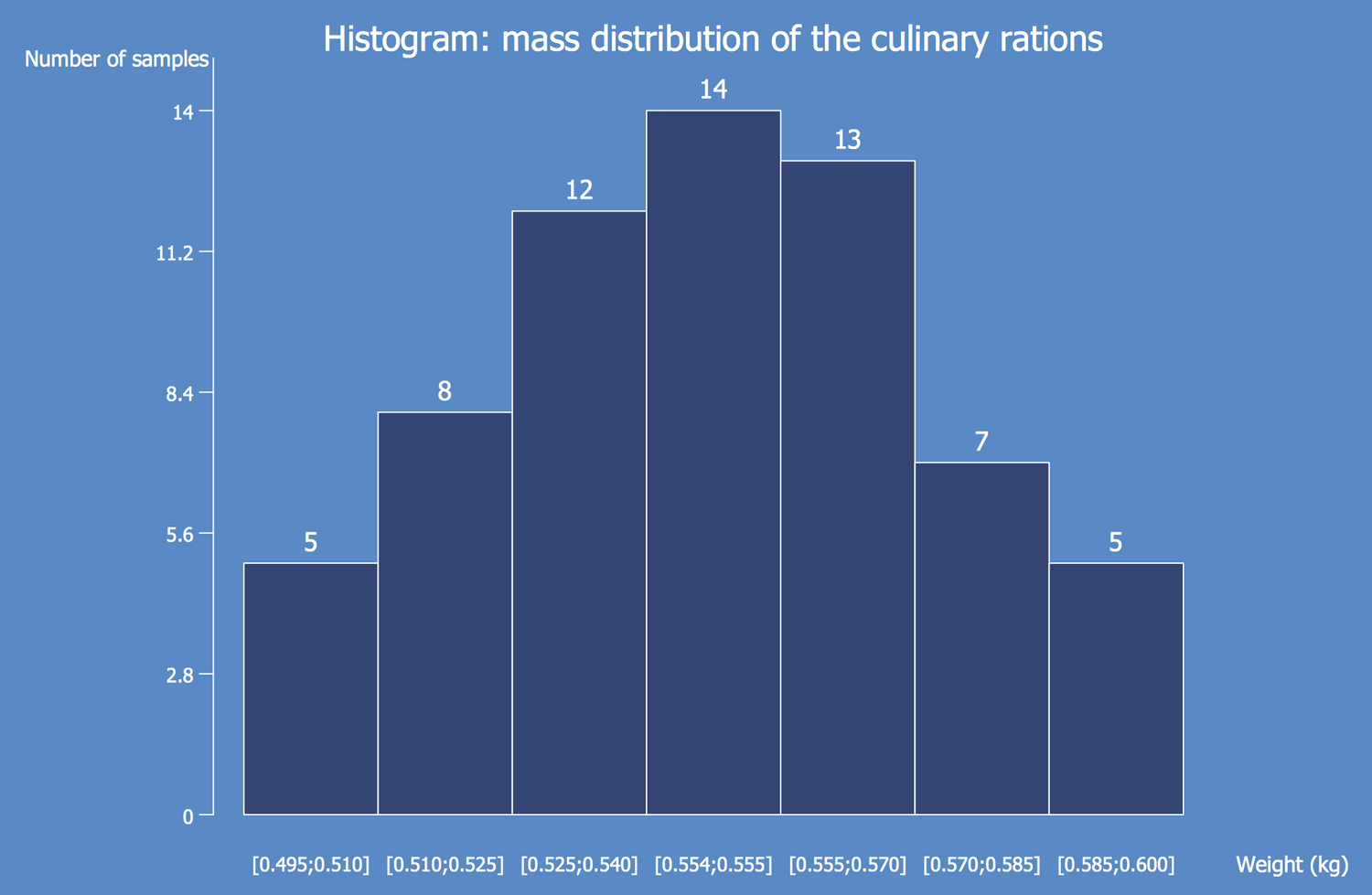## Sample 9: Mixture of Two Populations

Histograms sample: Mixture of Two Populations.

This example is created using ConceptDraw DIAGRAM diagramming software enhanced with Histograms solution from ConceptDraw Solution Park.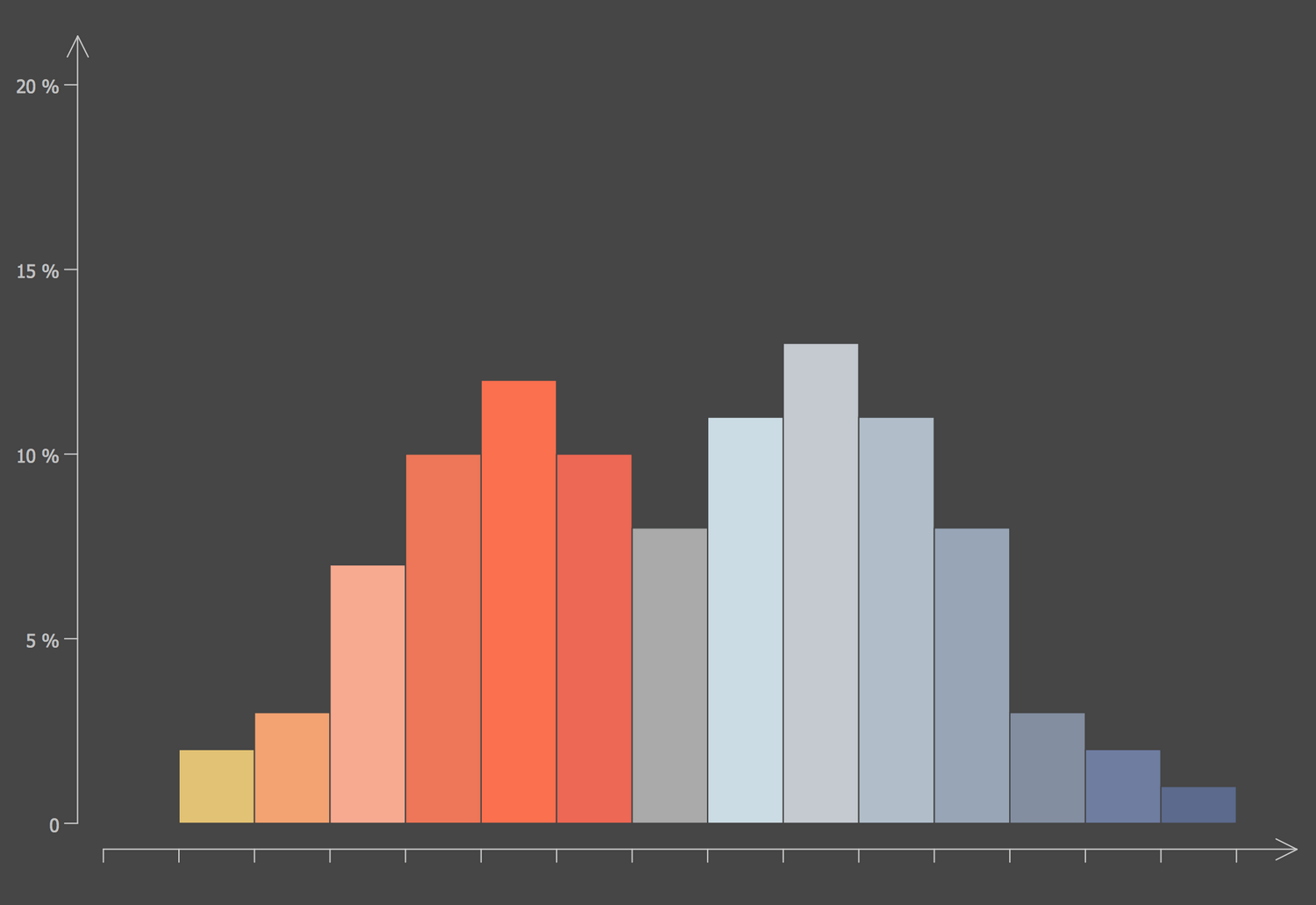## Sample 10: Normal Distribution

Histograms sample: Normal Distribution.

This example is created using ConceptDraw DIAGRAM diagramming software enhanced with Histograms solution from ConceptDraw Solution Park.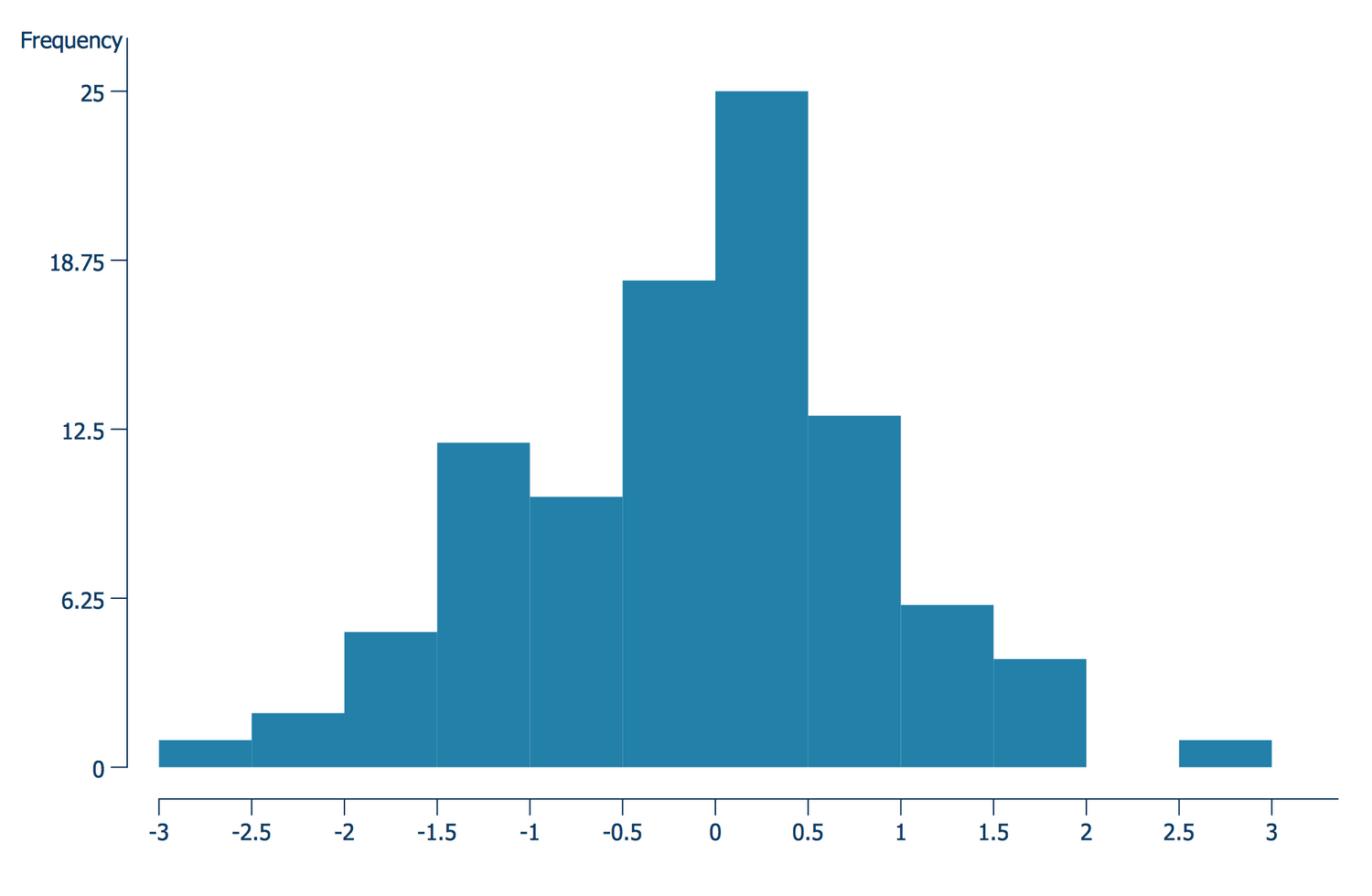## Sample 11: Repartition of Height

Histograms sample: Repartition of Height.

This example is created using ConceptDraw DIAGRAM diagramming software enhanced with Histograms solution from ConceptDraw Solution Park.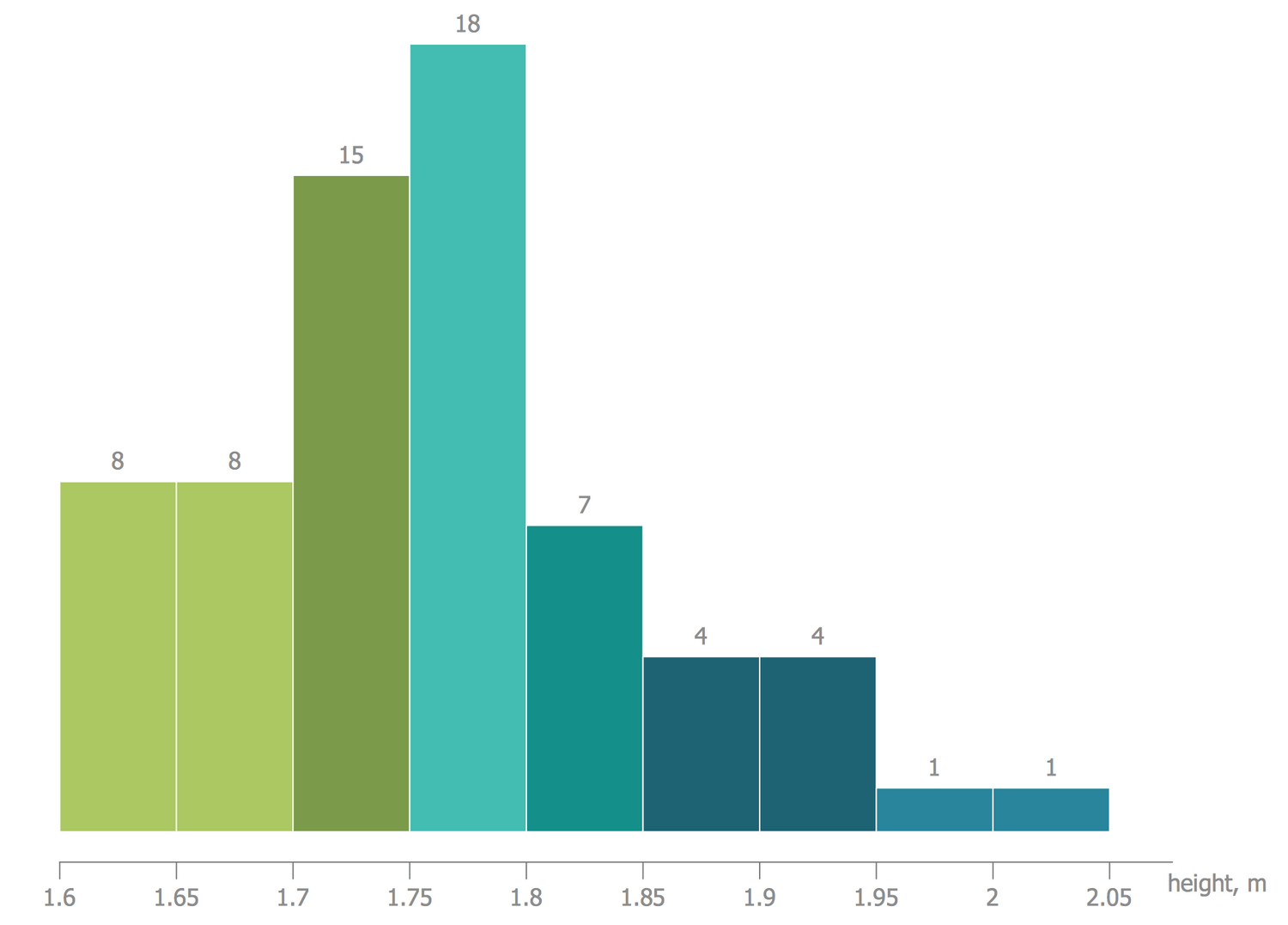All samples are copyrighted CS Odessa's.

Usage of them is covered by Creative Commons “Attribution Non-Commercial No Derivatives” License.

The text you can find at: https://creativecommons.org/licenses/by-nc-nd/3.0